The blocks A and B are arranged as shown in the figure. The pulley is frictionless. The mass of A is 10 kg. The coefficient of friction of A with the horizontal surface is 0.20. The minimum mass of B to start the motion will be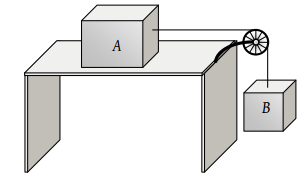(1) 2 kg

(2) 0.2 kg

(3) 5 kg

(4) 10 kg

Concept Questions :-

Types of forces
High Yielding Test Series + Question Bank - NEET 2020

Difficulty Level:

A uniform chain of length L hangs partly from a table which is kept in equilibrium by friction. The maximum length that can withstand without slipping is l, then coefficient of friction between the table and the chain is

(1) $\frac{l}{L}$

(2) $\frac{l}{L+l}$

(3) $\frac{l}{L-l}$

(4) $\frac{L}{L+l}$

Concept Questions :-

Friction
High Yielding Test Series + Question Bank - NEET 2020

Difficulty Level:

When two surfaces are coated with a lubricant, then they

(1) Stick to each other

(2) Slide upon each other

(3) Roll upon each other

(4) None of these

Concept Questions :-

Friction
High Yielding Test Series + Question Bank - NEET 2020

Difficulty Level:

A 20 kg block is initially at rest on a rough horizontal surface. A horizontal force of 75 N is required to set the block in motion. After it is in motion, a horizontal force of 60 N is required to keep the block moving with constant speed. The coefficient of static friction is

(1) 0.38

(2) 0.44

(3) 0.52

(4) 0.60

Concept Questions :-

Friction
High Yielding Test Series + Question Bank - NEET 2020

Difficulty Level:

A block A with mass 100 kg is resting on another block B of mass 200 kg. As shown in figure a horizontal rope tied to a wall holds it. The coefficient of friction between A and B is 0.2 while coefficient of friction between B and the ground is 0.3. The minimum required force F to start moving B will be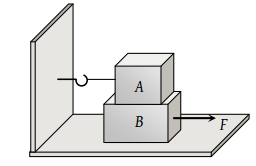(1) 900 N

(2) 100 N

(3) 1100 N

(4) 1200 N

Concept Questions :-

Friction
High Yielding Test Series + Question Bank - NEET 2020

Difficulty Level:

A horizontal force of 10 N is necessary to just hold a block stationary against a wall. The coefficient of friction between the block and the wall is 0.2. The weight of the block is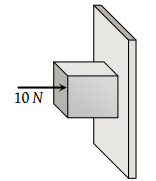(1) 2 N

(2) 20 N

(3) 50 N

(4) 100 N

Concept Questions :-

Types of forces
High Yielding Test Series + Question Bank - NEET 2020

Difficulty Level:

The coefficient of static friction, μs, between block A of mass 2 kg and the table as shown in the figure is 0.2. What would be the maximum mass value of block B so that the two blocks do not move? The string and the pulley are assumed to be smooth and massless. (g = 10 m/s2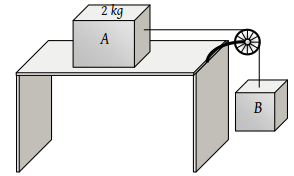(1) 2.0 kg

(2) 4.0 kg

(3) 0.2 kg

(4) 0.4 kg

Concept Questions :-

String constraint
High Yielding Test Series + Question Bank - NEET 2020

Difficulty Level:

If mass of A = 10 kg, coefficient of static friction = 0.2, coefficient of kinetic friction = 0.2. Then mass of B to start motion is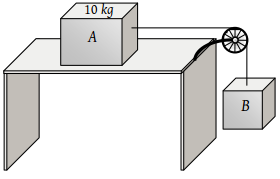(1) 2 kg

(2) 2.2 kg

(3) 4.8 kg

(4) 200 gm

Concept Questions :-

Application of laws
High Yielding Test Series + Question Bank - NEET 2020

Difficulty Level:

The maximum speed that can be achieved without skidding by a car on a circular unbanked road of radius R and coefficient of static friction μ, is

(1) $\mu Rg$

(2) $Rg\sqrt{\mu }$

(3) $\mu \sqrt{Rg}$

(4) $\sqrt{\mu Rg}$

Concept Questions :-

Friction
High Yielding Test Series + Question Bank - NEET 2020

Difficulty Level:

A car is moving along a straight horizontal road with a speed v0. If the coefficient of friction between the tyres and the road is μ, the shortest distance in which the car can be stopped is

(1) $\frac{{v}_{0}^{2}}{2\mu g}$

(2) $\frac{{v}_{0}}{\mu g}$

(3) ${\left(\frac{{v}_{0}}{\mu g}\right)}^{2}$

(4) $\frac{{v}_{0}}{\mu }$

Concept Questions :-

Friction